# KSEEB Solutions for Class 9 Maths Chapter 5 Triangles Ex 5.3

In this chapter, we provide KSEEB SSLC Class 9 Maths Chapter 5 Triangles Ex 5.3 for English medium students, Which will very helpful for every student in their exams. Students can download the latest KSEEB SSLC Class 9 Maths Chapter 5 Triangles Ex 5.3 pdf, free KSEEB SSLC Class 9 Maths Chapter 5 Triangles Ex 5.3 pdf download. Now you will get step by step solution to each question.

## Karnataka Board Class 9 Maths Chapter 5 Triangles Ex 5.3

Question 1.
∆ABC and ∆DBC are two isosceles triangles on the same base BC and vertices A and D are on the same side of BC. If AD is extended to intersect BC at P, show that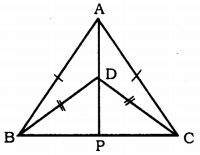(i) ∆ABD ≅ ∆ACD
(ii) ∆ABP ≅ ∆ACP
(iii) AP bisects ∠A as well as ∠D.
(iv) AP is the perpendicular bisector of BC.
Solution:
Data : ∆ABC and ∆DBC are two isosceles triangles on the same base BC and vertices A and D are on the same side of BC. AD is extended to intersect BC at P.
To Prove:
(i) ∆ABD ≅ ∆ACD
(ii) ∆ABP ≅ ∆ACP
(iii) AP bisects ∠A as well as ∠D.
(iv) AP is the perpendicular bisector of BC
(v) AD is the angular bisector of ∠A.
Proof:
(i) In ∆ABD and ∆ACD,
AB = AC (data)
BD = DC (data)
S.S.S. Congruence rule.
∴ ∆ABD ≅ ∆ACD

(ii) In ∆ABP and ∆ACP,
AB = AC (data)
∠ABP = ∠ACP (Opposite angles)
∠BAP = ∠CAP (∵ ∆ABD ≅ ∆ACD proved)
Now ASA postulate.
∆ABP ≅ ∆ACP.

AP bisects ∠A.
In ∆BDP and ∆CDP,
BD = DC (data)
BP = PC (proved)
DP is common.
∴ ∆BDP ≅ ∆CDP (SSS postulate)
∴ ∠BDP = ∠CDP
∴ DP bisects ∠D.
∴ AP bisects ∠D.

(iv) Now, ∠APB + ∠APC = 180° (Linear pair)
∠APB + ∠APB = 180°
2 ∠APB = 180
∴ ∠APB = 1802
∴∠APB = 90°
∠APB = ∠APC = 90°
BP = PC (proved)
∴ AP is the perpendicular bisector BC.

(v) AP is the angular bisector of ∠A.
Angular bisector of ∠A is aD, because AD, AP is in one line.

Question 2.
AD is an altitude of an isosceles triangle ABC in which AB = AC. Show that A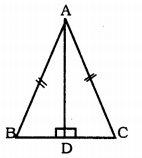Solution:
Data: AD is an altitude of an isosceles triangle ABC in which AB = AC.
To Prove:
Proof: i) In ∆ABD and ∆ACD,
AB = AC (data)
∴ ∆ABD ≅ ∆ACD
∴ BD = DC

Question 3.
Two sides AB and BC and median AM of one triangle ABC are respectively equal to sides PQ and QR and median PN of ∆PQR. Show that :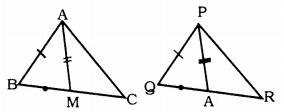(i) ∆ABM ≅ ∆PQN
(ii) ∆ABC ≅ ∆PQR.
Solution:
Data: Two sides AB and BC and median AM of one triangle ABC are respectively equal to sides PQ and QR and median PN of ∆PQR.
To Prove:
(i) ∆ABM ≅ ∆PQN
(ii) ∆ABC ≅ ∆PQR.
Proof: (i) In ∆ABC,
AM is the median drawn to BC.
∴ BM = 12 BC
Similarly, in ∆PQR,
QN = 12 QR
But, BC = QR
12 BC = 12 QR
∴ BM = QN
In ∆ABM and ∆PQN,
AB = PQ (data)
BM = QN (data)
AM = PN (proved)
∴ ∆ABM ≅ ∆PQN (SSS postulate)

(ii) In ∆ABC and ∆PQR,
AB = PQ (data)
∠ABC = ∠PQR (proved)
BC = QR (data)
∴ ∆ABC ≅ ∆PQR (SSS postulate)

Question 4.
BE and CF are two equal altitudes of a triangle ABC. Using the RHS congruence rule, prove that the triangle ABC is isosceles.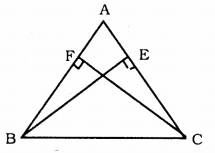Solution:
Data: BE and CF are two equal altitudes of a triangle ABC.
To Prove: ABC is an isosceles triangle.
Proof : BE = CF (data)
In ∆BCF and ∆CBE,
∠BFC = ∠CEB = 90° (data)
BC is a common hypotenuse.
As per Right angle, hypotenuse, side postulate,
∴ ∆BCF ≅ ∆CBE
∴ ∠CBF = ∠BCE
∴ ∠CBA = ∠BCA
∴ AB = AC
∴ ∆ABC is an isosceles triangle.

Question 5.
ABC is an isosceles triangle with AB = AC. Draw AP ⊥ BC to show that ∠B = ∠C.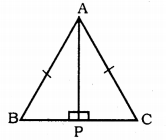Solution:
Data: ABC is an isosceles triangle with AB = AC.
To Prove : ∠B = ∠C
Construction: Draw AP ⊥ BC.
Proof: In ∆ABC, AP ⊥ BC and AB = BC.
∴ In ∆ABP and ∆ACP
∠APB = ∠APC = 90° ( ∵ AP ⊥ BC)
Hypotenuse AB = Hypotenuse AC
AP is common.
As per RHS Postulate,
∆ABP ≅ ∆ACP
∴ ∠ABP = ∠ACP
∴ ∠ABC = ∠ACB
∴∠B = ∠C.

All Chapter KSEEB Solutions For Class 9 Maths

—————————————————————————–

All Subject KSEEB Solutions For Class 9

*************************************************

I think you got complete solutions for this chapter. If You have any queries regarding this chapter, please comment on the below section our subject teacher will answer you. We tried our best to give complete solutions so you got good marks in your exam.

If these solutions have helped you, you can also share kseebsolutionsfor.com to your friends.

Best of Luck!!